3D Geometry Primer: Chapter 2 - Issue 01 - Points And Lines by (26 August 2002) Return to The Archives
 IntroductionIt must have been more than two years ago when I first contacted flipCode to ask if they were interested in posting some kind of mathematical tutorial series. We agreed about the format and about six months later (I write very slowly :), on the 14th of August 2000, the first issue was launched. When two months later chapter one was finished, the bonus was some interesting contacts and a flipShirt. It took me a very long time, but now I'm back for some more 3D excitement. In this chapter, I'll show you how to construct geometry in 3D space. In chapter I, we discussed vectors and how to represent points, but that aint much. How can we represent lines, planes, spheres, cylinders, pyramids, polygons and polyhedra? How can we work with them, for example how can we find out what's the intersection of a sphere and line? All this, we'll try to find out in this chapter.But before we start, I want to thank the following people without whom this second chapter wouldn't have been possible: Nicolas "Nick" Capens, for the incredible correction work that should make this stuff a hell of a lot easier to read, and for keeping my brain alive. Zhe Plateau rules! Yeah! Jaap "Jape" Suter, for writing the juggling tutorial and being Jaap. Oh yeah, and of course for his question "Wanneer ga je nou eindelijk een volgend hoofdstuk schrijven, man?" which translates to something like "Write a 2nd chapter, now!" ;) Freddy "de Freddie" Allemeersch, for introducing me to the most incredible world of 3D geometry, already 6 years ago now ("Aha, den super truck!"). Max McGuire for defending the crazy thoughts of a crazy author. He knows what I'm talking about :) Per "psykotic" Vognsen for helping a silly author out of his mess. He also knows it. Jarkko "Altair" Lempiainen for helping me on the C++ part of this chapter. Thanks for sharing your so appreciated guru knowledge :) Of course also Jacco "the Phantom" Bikker, Willem H. de Boer, Conor "DirtyPunk" Stokes, Thomas "lycium" Ludwig, Brecht "Palsy Palooka" Ameije, Lode "Hybrid" Vandevenne, Kurt Miller, Maarten Cauwe, Pascal Lotiquet, Pieter Devolder, Bart Van Haecke, Kris de Greve, Lode Ameije, Bram Lycke, Mathias Vandeputte, Pieter Nechelput, Wim Hogie, Dries Aelter, Maarten Deceuninck, and everybody else at KSA Rooyghem (I just don't feel to write all these names out, there are just too many :) for their great support and being who they are. Everybody who reads this for being interested in the column. Everybody who doesn't read this to avoid wasiting any bandwidth. Everybody who hates me (They always say to keep your friends close, but you're enemies closer. I don't know if that also means you need to thank them, anyway, who cares? :) And everybody else too. Merci w�!"Nine out of ten people like chocolate ... The tenth person always lies.", John G. Tullius, American artist and cartoonistAnd now, all things start small: back to points ...

 (I) Representation of 3D PointsAs we all already know, we simply use position vectors to represent points, to store them in memory (Position vectors are what I used to call place vectors, but it seems that this wasn't proper English :). That means we need an origin O and a (right handed) vector base B=. The position vector OP is the vector that fits between the origin O and the point P in question. The vector base is used to convert the position vector OP to the numerical information (x, y, z), in such way that OP = x*I + y*J + z*K. If you don't understand this, then maybe you should check out chapter I first.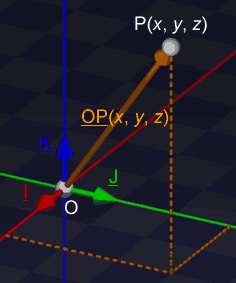Some people (especially Jaap ;) will now say: is this the guy who didn't want to use vector classes to store points or vice versa? Yes he is! And he still doesn't want to because he thinks it's a bad practice of OOP. Look, maybe this is nitpicking, but it's very logical if you want to understand it. And if to you 3D geometry is one misty world, then you should nitpick at this. In the end, you'll understand things better. So, I'll try to explain it once more:Vectors are one class of objects and you can do all sorts of stuff on it: addition, scaling, normalizing, cross products, dot products, etc., etc. ... Points are another class of objects. You can't scale points, you can't normalize them, nor you can calculate the cross or dot product of two points. But you can calculate the distance between two points, and that's something you can't do with vectors.To store a point in memory we use a position vector. That does *not* mean that points are position vectors! When we do operations on points, we'll do an equivalent operation on its position vector. That does *not* mean that all operations that can be done on vectors can be done on points and vice versa!You can store a line in memory by storing a couple of points as we'll see in a minute. Does this mean that a line is-a couple of points? No, it isn't. Does it mean that a couple of points is-a a line? No, it isn't. A line is much more than that! It is a set of an infinite number of points, not just those two stored in memory. The same thing counts for points: the position of the point is stored by a vector, but the point isn't the vector.This reflects very simply in OOP: a vector has properties a point doesn't have, and a point has properties a vector doesn't have. That means they can't be one and the same thing. You can't even derive one from the other. The derived class would inherit properties of the base class which it shouldn't inherent because it doesn't have them! The only way to implement points in terms of vectors is by containment, and the most logical choice is to let a point contain a vector: it's position vector.

 (II) Possible Relationships Between PointsWhat can we say about the relationship between two points? Very simple: two points are equal or they're not. Equal or not equal, that's the question! We call two points that are equal coincident points.coincident points = identical points = equal points non-coincident points = different points

 (III) Distance Between 2 PointsI guess most of you already know how to use Pythagoras' theorem for this. But why can we use it? I'll explain this in a simple, but quite elegant way ...Say we have two points P(p1, p2, p3) and Q(q1, q2, q3), and we want to know dist(P, Q), the distance between them. How? What we can do is pull a vector PQ between these points: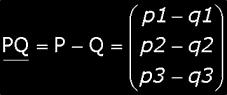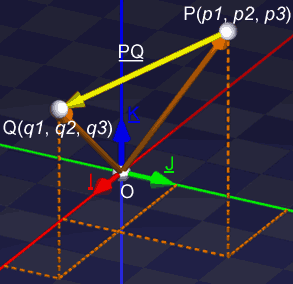You can see that the length of this vector PQ will be exactly the distance between P and Q. Wonderful huh? So, all we have to do to know this distance is calculate the magnitude of PQ.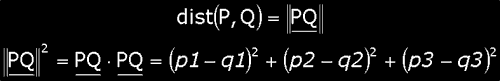and at the end, we get exactly Pythagoras' formula: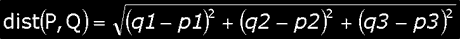(IV) Representation Of Lines: Parametric EquationsA line is actually a infinitely large set of points, and all these points lie on (be surprised!) one straight line. To store a line d (I'll be using bold non-italic small letters for lines), we could save all these points; and yes, we would have represented our line unambigously. However, there are infinitely many points on every line, and no computer memory can handle that. So, we need to use a trick ...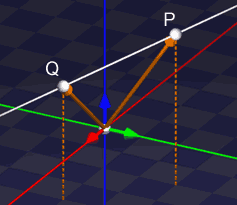We all know that we can draw exactly one line through two different points (remark 1). Two different lines can only have 0 or 1 points in common. So if we have two points, you can find only one line that goes through these points. If you would find another, than both lines would have more than one point in common. This means both lines are identical and thus it is contradictory that you have found a different one ...Well, this is not exactly true: two lines d and e can have 0 or 1 or all their points in common. However, if they have all points in common, then they are in fact the same line. A little confusing maybe.This means that if we take two different points of our line, we have completely represented our line. And now we can say: "OK, to store that line, we simply store two different points of that line and that's it. We've stored our line unambigously". While this is true and can be stored in a finite amount of memory, there's even a better way ...a) Direction VectorConsider one point as the support point of the line. You can see this point as the one that carries the line, the one that holds the line at its position. Any point of the line can be used for this job, but you only need one. So you simply pick one. We call that point S, and pull vectors to all other points of the line. Now you're able to reach each point of the line, by adding such a vector to S. "Wait a minute, what are you trying to say?"Take the line d with support point S and one extra point P. And out of the blue, pull a vector V between S and P. We now know that the positions S and P are actually stored by vectors OS and OP respectively. Thus, of V we know that V = SP = P � S = OP � OS.Now, consider the situation where we only know S and V, and that we want to reach (= to know) the point P. To do this, we only have to add V to S, and we become P. i.e. P = S + V, or OP = OS + V. I think this is quite clear, but do you realize what it means? From the moment we have a support point S, and all the vectors along the line d, we will be able to reach each point of the line, by adding such a vector to S.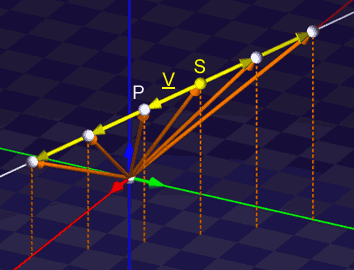"OK, but you still need a point S and infinitely many vectors. How do you store *that*?" Aha, don't you see that all these vectors have the same direction (not always the same sense, however)?. This means that all these vectors can be written as a scalar multiplication of only one vector D: for each vector V on line d, you will be able to find a real number t, so that V = t*D.Eventually, you find that each point P of the line, can be written as P = S + t*D, or with position vectors: OP = OS + t*D. This is called the parametric equation of the line, with t as the parameter. Notice that this equation only uses 2 vectors to find all the points of the line: you only need to store S and D to store your line! (Remember that we use the position vector OS to store the position of point S.)We call this vector D the direction vector: the direction of d is the same as the direction of D. You can use any vector as the direction vector, as long as it is parallel to the line (though, you can't choose the null vector, since it's direction is undefined). So, just choose one as D. In a minute, we'll see that things become a lot easier if D is normalized: i.e. ||D|| = 1 or D is a unit vector.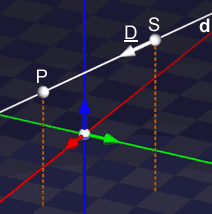P = S(2, 3, 4) + 3 * D(2/3, -2/3, -1/3) = (4, 1, 3)Anyway, a line d with support point S and direction D, has the following parametric representation: P = S + t*D with t � ]��, +�[. For each parametric equation you can say that it generates points of the object, of a line in this case. If you substitute the parameter (e.g. t=5), then you find a point of the object. For each value of the parameter, you will find another point. e.g. if S=(2, 3, 4) and D=(2/3, -2/3, -1/3), and you substitute t=3, you will generate the point P = (2, 3, 4) + 3 * (2/3, -2/3, -1/3) = (4, 1, 3).You can write the same parametric equation in many ways. The following are all the same equations: they all represent the same line d with support point S(s1, s2, s3) and direction vector D(d1, d2, d3). They only differ in the way they're written.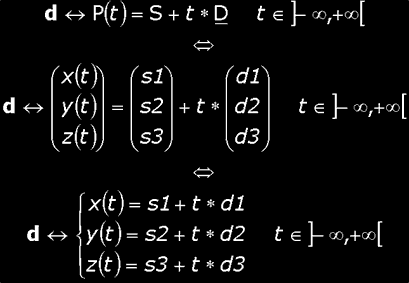Notice that each line mentions the interval of the parameter t: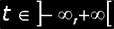This is to make clear that d indeed represents a line. d is indeed the set of all points P(t) for t going from �� to +�.b) Barycentric CombinationsAlthough I've said that storing a line by a support point S and direction vector D is better than just storing 2 points of the line, I'll show you now that the latter is also possible and sometimes more elegant.Consider you have defined your line through 2 points S and R. You choose S to be the support point and you construct the direction vector D = R � S. Now you have your equation P = S + t*D. If you want to use R and S to express your line, the only thing you have to do is to substitute D by what it means: R � S. In this way you get a new equation: P = S + t*(R � S) = (1 � t)*S + t*R. Officially you get: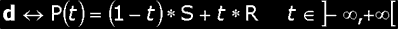This new equation is called the barycentric parametric equation of the line. Why? Simple, each point P is expressed by the barycentric combination of points S and R with coefficients (1 � t) and t respectively. Notice that the sum of the coefficients (1 � t) + t = 1, which is required for barycentric combinations.c) Line SegmentsBarycentric equations are a nice way to represent the line segment [SR] between points S and R. If you fill in t = 0, you will get S; for t = 1, you will get R and for every other value between 0 and 1, you will get a point between S and R. Compare this with the definition of a convex barycentric combination: if all coefficients are positive, then the barycentric combination is in the convex hull (which is the line segment in case of 2 points). Here these coefficients are positive if and only if t � [0, 1].In this way, you can define the line segment [SR] as like following: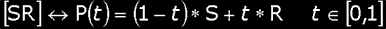You can check out more information about barycentric combinations here. Especially check out example (c) in paragraph (iv). Does it look familiar to you?d) Orientation Of A LineGenerally lines are not oriented. If you are the support point S, you can look along the line in two different ways: to one end or the other. If a line isn't oriented, both ends are equally important. There's no discrimination.However, if you use a line to represent a light ray, you don't want that. This light ray starts at a certain point (the source) and then it propagates in only one direction and sense. And what's more: for every two points of this ray, you can say which one is further away (from the source) than the other one.Fortunately, we already have the solution. Look at the parametric equation, put the support point S at the light source and point the direction D in the way you want the light ray to go. Now you will find out that the points you want to be part of the ray, are the points with a positive t value. And the bigger the value t, the further it's away from the source.More generally, an oriented line is a line of which you define one end to be positive, and the other to be negative. This can easily be stored in the computer by choosing the direction vector in such a way that it points to the positive end of the line.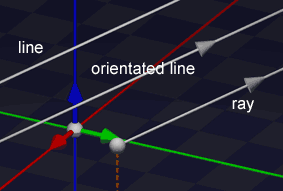Note: With "the ends of the line", I meant the points at infinity; but it works the same way for line segments.

 (V) Cartesian Equation Of A LineNow we know how to represent a line with parametric equations. But that's not the only way to do it. You can also use cartesian equations. A cartesian equation of something, is per definition an equation of that object without any parameter. This means that it can't generate points of the object like a parameter equation does. What it can do however, is allow you to check if a certain point is part of the object. e.g.: you fill in a point P=(4, 1, 2), and if the equation is true the point is part of the object, else it is not. This is confusing? I'll show you how it's done in the case of a line.We have the parametric equation and we want the cartesian one. So we need a way to transform the former into the latter. The obvious way to do this, is to get rid of the parameter: you need to eliminate the parameter t.First, look at the parametric equation as three different equations: one for the x-, one for the y- and one for the z component.Next, you separate t in each equation: i.e. you put t at the left, and everything else to the right. You get the following things (check out if you can get there yourself):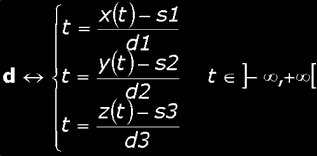Notice how each of these equations contains the *same* t. This is very important! If you fill in t (e.g. t = 5) for one of these equations, you *must* fill in the same value for the other two! And that's what we're going to use to eliminate t. We're going to demand that these t's are the same value: say t = t = t. And now you substitute each t by one of the 3 equations. i.e. you replace each of these t's by the right expression of each line. Then you get: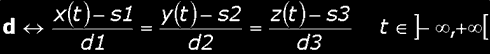and without the explicit t dependency (what we want) we get:And this is our cartesian equation! Wow! If you fill in S(s1, s2, s3) = (2, 3, 4), D(d1, d2, d3) = (2/3, -2/3, -1/3) and P(x, y, z) = (4, 1, 3); then you will find out that this equation is indeed true. You will find: 3 = 3 = 3. We get back our original t! Superb!I don't know many cases where the cartesian equation of a line is used. Mostly one prefers the parametric equation. It's hard to explain why. It's just because that one makes problems easier to solve. We'll even see that in the case of a plane, you will rather use the cartesian one instead of the parameter equation. Odd huh?That's it for this week since this issue is already getting longer then I've intended to. Next week we're going to learn what the different relations between lines are, how we can calculate the distance between a point and a line, and some other things.Special thanks to Nick for correcting this stuff.Stay tuned! Bramz

 Remarks(1) Well, this is not exactly true: a line can have none, exactly one or all of its points in common with another line. However, if two lines have all points in common, then they are in fact the same line. We call that coincident lines. A little confusing maybe.